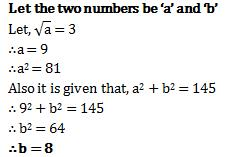Courses

# Test: Squares And Square Roots- 1

## 10 Questions MCQ Test Quantitative Techniques for CLAT | Test: Squares And Square Roots- 1

Description
This mock test of Test: Squares And Square Roots- 1 for Quant helps you for every Quant entrance exam. This contains 10 Multiple Choice Questions for Quant Test: Squares And Square Roots- 1 (mcq) to study with solutions a complete question bank. The solved questions answers in this Test: Squares And Square Roots- 1 quiz give you a good mix of easy questions and tough questions. Quant students definitely take this Test: Squares And Square Roots- 1 exercise for a better result in the exam. You can find other Test: Squares And Square Roots- 1 extra questions, long questions & short questions for Quant on EduRev as well by searching above.
QUESTION: 1

### Which of the following is a perfect square number?

Solution: The answer is 1681 because it is the only number which has it's last digit as a number which a perfect square can have . 9×9=81 the last digit is 1.
QUESTION: 2

### Which of the following would end with digit 1?

Solution: Option B is correct because the unit digit of 161 is 1 and if unit digit of any digit ends with 1 the its square will also end with 1.
QUESTION: 3

### Without adding, find the sum. 1 + 3 + 5 + 7 + 9

Solution:
Here, we have to find the sum of first five odd natural numbers.
Therefore, 1 + 3 + 5 + 7 + 9 = (5)2 = 25
QUESTION: 4

Find the smallest whole number by which 1008 should be multiplied to get a perfect square number .

Solution:

QUESTION: 5

When a square number ends in _______, the number whose square it is, will have either 4 or 6 in unit’s place.

Solution: Answer is 6 because the last digit of the square of 4 is 6 and 4 is also 6.
QUESTION: 6

Sum of squares of two numbers is 145. If square root of one number is 3, find the other number.

Solution:QUESTION: 7

Find the perfect square numbers between 30 and 40.

Solution:
Since, 1 x 1 = 1
2 x 2 = 4
3 x 3 = 9
4 x 4 = 16
5 x 5 = 25
6 x 6 = 36
7 x 7 = 49

Thus, 36 is a perfact square number between 30 and 40.
QUESTION: 8

All square numbers end with __________ at unit’s place.

Solution:

The numbers ending with 0 gives 0,numbers ending with 1,9 gives 1, numbers ending with 2,8 gives 4, numbers ending with 3,7 gives 9, numbers ending with 5 gives 5 and numbers ending with 4,6 gives 6. Hence we get only 0,1,4,5,6,9 at the unit's place.

QUESTION: 9

Without doing any calculation, find the numbers which are surely perfect squares.

Solution:
QUESTION: 10

How many numbers lie between square of 12 and 13

Solution:

122 = 12*12 = 144

132 = 13*13 = 169

Now numbers are between144 and 169 are:

145, 146, 147,.............168

Total number = 24

So total numbers lies between 144 and 169 is 24